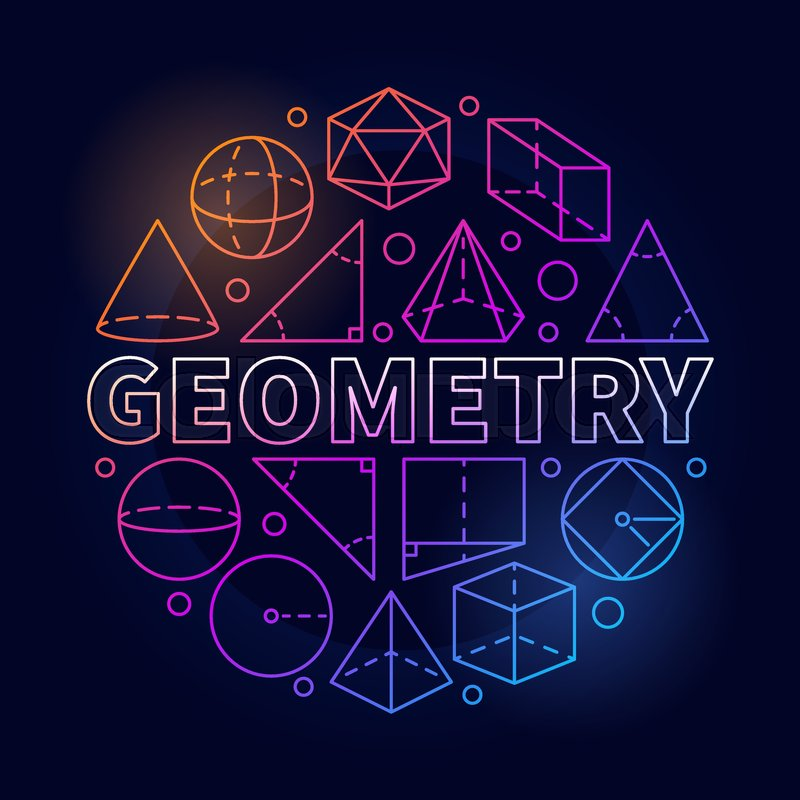# Geometry II

Welcome to Class !!

In today’s Mathematics class, We will continue our lesson on Geometry. We hope you enjoy the class!#### GEOMETRY CONT’D

Areas and Volumes of similar shaped

Scale Factor (Length Ratio)

P          9cm              Q

A                  B

2                                         6cm

D    3          C

S                                    R

Area factor   = (Scale factor)2

If the ratio of the length is X: Y

Then the ratio of the area is  X2: Y2

Example 1:

In the diagram below, if the area of triangle ABC is 48cm2, find the area of triangle XYZ to 3s.f

A                                         X

550

650       600                                                    600

B        12cm         C            Y      4cm       Z

Solution

In ∆ABC,  <A   = 180 – (65 + 60)  = 55

In ∆XYZ, <Y  = 180 – (55-60) = 650

Since then corresponding angles are equal, ∆ABC and ∆XYZ are similar

#### The volume of Similar Shapes

Volume factor = (Scale factor)3

If the ratio of the length is X: Y

Then the ratio of the volume is X3: Y3

Example 2: The ratio of a cylinder of volume 2970cm3 is 30mm. find the volume of a similar cylinder of radius 40mm.

Solution

We have come to the end of this class. We do hope you enjoyed the class?

Should you have any further question, feel free to ask in the comment section below and trust us to respond as soon as possible.

In our next class, we will be talking about the Area of Plane Figures. We are very much eager to meet you there.

How Can We Make ClassNotesNG Better? - CLICK to Tell Us Now!